# JP5242355B2 - Electric heat cogeneration energy system - Google Patents

## Info

Publication number
JP5242355B2
JP5242355B2 JP2008310992A JP2008310992A JP5242355B2 JP 5242355 B2 JP5242355 B2 JP 5242355B2 JP 2008310992 A JP2008310992 A JP 2008310992A JP 2008310992 A JP2008310992 A JP 2008310992A JP 5242355 B2 JP5242355 B2 JP 5242355B2
Authority
JP
Japan
Prior art keywords
heat
electric
means
electric heat
amount
Prior art date
Legal status (The legal status is an assumption and is not a legal conclusion. Google has not performed a legal analysis and makes no representation as to the accuracy of the status listed.)
Expired - Fee Related
Application number
JP2008310992A
Other languages
Japanese (ja)
Other versions
JP2010136557A (en
Inventor

Original Assignee

Priority date (The priority date is an assumption and is not a legal conclusion. Google has not performed a legal analysis and makes no representation as to the accuracy of the date listed.)
Filing date
Publication date
Application filed by 株式会社東芝 filed Critical 株式会社東芝
Priority to JP2008310992A priority Critical patent/JP5242355B2/en
Publication of JP2010136557A publication Critical patent/JP2010136557A/en
Application granted granted Critical
Publication of JP5242355B2 publication Critical patent/JP5242355B2/en
Application status is Expired - Fee Related legal-status Critical
Anticipated expiration legal-status Critical

## Images

•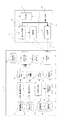•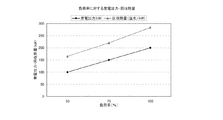••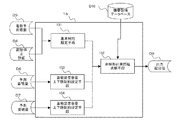•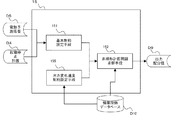•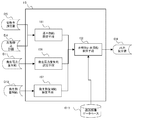•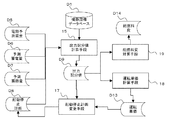•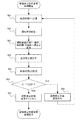•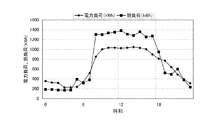•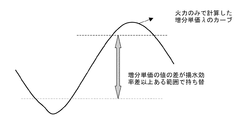•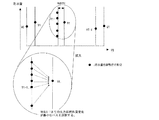## Classifications

• YGENERAL TAGGING OF NEW TECHNOLOGICAL DEVELOPMENTS; GENERAL TAGGING OF CROSS-SECTIONAL TECHNOLOGIES SPANNING OVER SEVERAL SECTIONS OF THE IPC; TECHNICAL SUBJECTS COVERED BY FORMER USPC CROSS-REFERENCE ART COLLECTIONS [XRACs] AND DIGESTS
• Y02TECHNOLOGIES OR APPLICATIONS FOR MITIGATION OR ADAPTATION AGAINST CLIMATE CHANGE
• Y02PCLIMATE CHANGE MITIGATION TECHNOLOGIES IN THE PRODUCTION OR PROCESSING OF GOODS
• Y02P80/00Climate change mitigation technologies for sector-wide applications
• Y02P80/10Efficient use of energy
• Y02P80/15On-site combined power, heat or cool generation or distribution, e.g. combined heat and power [CHP] supply

## Abstract

<P>PROBLEM TO BE SOLVED: To improve system efficiency by enabling simultaneous optimization of both a power storage device and a heat storage device. <P>SOLUTION: A cogeneration energy system includes: an electric heat generating device 21 having both a power generation function and a heat generation function; and either of the power storage device 22 to store at least electric power generated by the electric heat generating device 21 and a heat generation device 23 to store heat generated by the electric heat generating device 21. In the cogeneration energy system, there are provided with: an electric heat demand predicting means 11 for predicting an electric heat demand of the overall energy system; a power storage amount predicting means 12 for predicting the power storage amount; a heat storage amount predicting means 13 for predicting the heat storage amount; and a starting/stopping plan preparation means 14 for preparing a starting/stopping plan of the electric heat generating device. Furthermore, the cogeneration energy system includes an output distribution value calculation means 15 for determining an output distribution value of the electric heat generating device 21 existing in the energy system, based on the predicted electric heat demand, the power storage amount, the heat storage amount and the starting/stopping plan so that the energy supply cost of the overall energy system is minimized by using a non-linear planning problem solving means. <P>COPYRIGHT: (C)2010,JPO&amp;INPIT

## Description

The present invention relates to an electric heat generator, and an electric and heat cogeneration energy system including at least one of a power storage device and a heat storage device connected thereto.

In recent years, in response to demands for environmental protection and energy saving, there is an increasing number of cases of introducing so-called combined electric and heat supply systems that recover exhaust heat from power generation and power generation facilities, convert it into heat suitable for usage, and supply it with electric power. . The cogeneration system aims to achieve extremely high overall efficiency by cascading primary energy from high quality to low quality. However, since heat supply is performed simultaneously with power generation, the above-mentioned high overall efficiency is basically based on the condition that the demand situation for power and the demand situation for heat coincide in terms of quantity and time. is there.

However, in actuality, the demand situation for power and the demand situation for heat do not necessarily match (showing the graph of power demand and heat demand in the region in FIG. 8), so the supply was matched to the demand for either power or heat. In such a case, a surplus arises, resulting in a problem that the overall efficiency as originally expected cannot be obtained. Therefore, measures are taken to ensure high overall efficiency by introducing power storage devices or heat storage devices.

Therefore, a problem in a system in which a power storage device or a heat storage device or both are introduced is when charging for a power storage (heat storage) device (heat storage) or power storage (heat storage) when considering operation in a certain period. Is it reasonable to discharge (heat radiation) from the device? When considering the operation of a power storage (heat storage) device for a certain period, due to the limitation of the capacity of the power storage (heat storage) device, it cannot continue to be unilaterally discharged (heat radiation), and it will be discharged (heat radiation) at a certain time zone. Since the amount of stored electricity (heat storage) is reduced, it is necessary to charge (store heat) in another time zone and recover the amount of stored electricity (heat storage).
In the conventional energy system control method, for example, with respect to the power storage device, it is usual to determine the time zone for charging at night and the time zone for discharging at the peak of the power load to operate in a rule. In addition, there has been no attempt to achieve optimum operation considering the power storage device and the heat storage device at the same time.

By the way, as an approach to this problem, an analogy from the operation of a pumped storage power plant in the power system can be considered. Regarding the operation of a pumped storage power plant, the following methods of operation have been proposed.

(Operational calculation of pumped storage power plant)
Method 1 : Change-over calculation considering the difference in pumping efficiency.
Method 2 : Calculate the pumped-storage generator in the same row as the thermal power generator. In other words, the water consumption characteristics of the pumped-storage generator are assumed to be equivalent to the thermal power consumption characteristics of the thermal power, and are simulated to simulate a thermal power machine with an output range extended to minus (water / fire cooperative calculation).
Method 3 : Optimize water storage. That is, the change in the amount of stored water is replaced with the change in fuel cost of thermal power, and the amount of stored water that minimizes the change in fuel cost is obtained by dynamic programming.

As shown in FIG. 9, Method 1 is a method in which pumping and pumping are performed in consideration of the efficiency difference when the difference in incremental fuel costs is equal to or greater than the efficiency difference as a result of calculation only with the thermal power plant. Although it is the simplest method, the amount of stored water varies depending on the order of changeover, and there is no guarantee that the optimal solution will be obtained.

For Method 2, the concept of incremental water unit price is introduced, and the lambda method is calculated by calculating the following water / fire coordination formula at each time. May be undesirable in terms of numerical characteristics.

In Method 3, since the change in the amount of stored water causes a change in the thermal power sharing load, as shown in FIG. 10, the change in the amount of stored water is associated with the change in the thermal power cost, and the change in the thermal power cost is minimized (usually a negative value). The change in water storage is calculated by dynamic programming.

Although this method 3 is an effective method that can handle the upper and lower limits of the stored water amount at the same time and does not require the use water amount characteristic, the change in the stored water amount is expressed in discrete values (for example, in increments of 5 MWh). If you try to apply it to the problem that considers both the power storage device and the heat storage device like this problem, it will solve the multidimensional dynamic programming problem. This increases the calculation time.

In addition, as a method for controlling the power storage device in real time, as in the fuel cell system control method described in Patent Document 1, the energy cost is controlled in consideration of the power generation cost and the storage battery discharge cost. However, simultaneous optimization with the heat storage device has not been implemented.
JP 2003-163013 A Iwanami Lecture Applied Mathematics 15 [Method 7] Optimization Method Hiroshi Fujita, Hiroshi Konno, Kunishi Tabuchi (1998)

In the conventional energy system control method, power generation patterns and storage / discharge schedules that are considered to be economical with respect to known load patterns are determined in advance, and cannot cope with various load patterns of electric heat. It was. Moreover, the method based on the analogy from the operation method of the pumped storage power plant in the power system is not sufficient for the problem of simultaneously considering power and heat as described above.
The present invention has been made in view of the above problems, and an object of the present invention is to determine an optimum output distribution value that improves overall efficiency in an electric and cogeneration energy system having at least one of a power storage device and a heat storage device. It is said. Another object of the present invention is to provide a combined electric and heat energy system capable of simultaneously optimizing the power storage device and the heat storage device by handling the power storage device and the heat storage device under the same constraints.

In order to solve the above-described problems, the present invention provides an electric heat generation device having both a power generation function and a heat generation function, a power storage device that stores electric power generated by the electric heat generation device, and a heat storage device that stores heat generated by the electric heat generation device. In an electric heat supply energy system having at least one of the following, the electric heat demand prediction means for predicting the electric heat demand of the entire energy system, the storage amount prediction means for predicting the amount of electricity stored in the electricity storage device, and the heat storage device At least one of the heat storage amount prediction means for predicting the amount of stored heat and the start / stop plan creation means for creating a start / stop plan for the electric heat generator, and at least the predicted electric heat demand, the amount of stored electricity and the amount of heat storage On the other hand, based on the start / stop plan, the output distribution value of the electric heat supply in the electric heat generator is calculated using the non-linear programming problem solving means. Energy supply costs of the overall energy system is characterized by having an output distribution value calculating means for determining that a minimum.

In this case, the output distribution area calculation means includes the power supply / demand balance and heat demand balance acquired from the demand prediction means, the charge / discharge amount balance acquired from the storage amount prediction means, and the storage / release acquired from the heat storage amount prediction means. The fuel function minimization condition is an objective function with at least one of the heat balance, at least one of the simultaneous charging / discharging load constraint and the simultaneous heat storage / dissipation load constraint acquired from the start / stop plan creating means, and the load factor upper / lower limit constraint as constraints. As described above, it is preferable to obtain an optimal solution by means of nonlinear programming problem solving means.

By implementing the present invention, it is possible to provide a supply and demand control method for a combined electric and heat energy system that enables simultaneous optimization of the power storage device and the heat storage device and improves the overall system efficiency including the electric heat generation device.

According to the energy system control method of the present invention, the electric energy demand of the entire energy system, the start / stop plan (start / stop information of each device), the storage amount prediction value of the power storage device, and the heat storage amount prediction value of the heat storage device The output distribution value with the minimum total fuel cost of the electric heat generating device, power storage device and heat storage device over a plurality of time zones is calculated and controlled.

Embodiments of the present invention will be described with reference to the drawings.
FIG. 1 is an overall view of an energy system to which the first to fifth embodiments of the present invention are applied, and shows the connection between the energy system control device and each device, and the flow of power, heat and control information. . That is, the energy system control device 1 is connected to the electric heat generation device group 21, the power storage device 22, the heat storage device 23 through the control signal transmission means 31.

The power load 24 is supplied from the electric heat generating device group 21 or the power storage device 22. Further, the heat load 25 is supplied from the electric heat generating device group 21 or the heat storage device 23.
Information for controlling each device is transmitted from the supply and demand control device 1 to each device using the control signal transmission means 31. In addition, information on the operation state is transmitted to the energy system control device 1 from each device of the electric heat generation device group 21, the power storage device 22, and the heat storage device 23.

The energy system control device 1 is provided with an electric heat demand result database D1 in which electric heat demand results are stored by season, month, and day of the week. For example, a future electric heat forecast demand D5 is created.

The energy system control device 1 includes a storage amount result database D2 in which the storage amount results are stored by season, month, and day of the week. The storage amount prediction unit 12 includes a storage amount result database D2 and weather prediction information. Etc. is used to create a predicted power storage amount D6 in the future.
The energy system control device 1 includes a heat storage amount result database D3 in which the heat storage amount results are stored by season, month, and day of the week. The heat storage amount prediction means 13 includes a heat storage amount result database D3 and weather prediction information. For example, a future predicted heat storage amount D7 is created.

The energy system control device 1 includes an operation result database D4 in which operation results (particularly start / stop 0-1 information) of each electric heat generator are stored for each season, month, and day of the week. The means 14 creates a future start / stop plan D8 by using the operation result database D4, the predicted electric heat demand D5, the predicted power storage amount D6, the predicted heat storage amount D7, the weather prediction information, and the like. This start / stop plan may be set by a human system.

The output distribution value calculation means 15 is based on the predicted future electric heat demand D5, the predicted power storage amount D6, the predicted heat storage amount D7, and the start / stop plan D8. D9 is calculated (including the timing and amount of power storage and heat storage).
The device control means 16 transmits information for controlling each device based on the output distribution value D9 to the electric heat generating device group 21, the power storage device 22, and the heat storage device 23 via the control signal transmission means 31.

(First embodiment)
A first embodiment will be described.
In the present embodiment, the output distribution value calculation means 15 calculates each time of each device in a certain future period based on the predicted electric heat demand D5, the predicted power storage amount D6, the predicted heat storage amount D7, and the start / stop plan D8. The output distribution value (including the timing and amount of power storage and heat storage) D9 in the belt is calculated. In the present invention, the power distribution device and the heat storage device are utilized to determine the output distribution value with the minimum fuel cost.

Usually, in the electric heat generator, as shown in FIG. 2, both the power output and the heat output with respect to the load factor change linearly. Therefore, when the load factor is ν, the power output and the heat output can be expressed as follows.

In the following, d and e are called power output characteristic coefficients, and f and g are called heat output characteristic coefficients.
In the present embodiment, the power storage device is simulated by a set of a device dedicated to power storage (charging) and a device dedicated to discharging. The upper limit output PC max at time slot t of the power storage apparatus dedicated, and t, the maximum output at the time zone t of the discharge apparatus dedicated to the PD max, t, and the power load DP t at time zone t. Also, when the calculation target period is T s through T e, the power supply-demand balance constraints at any time period t in this embodiment can be expressed by Equation (1.2).

Here, the meaning of each symbol is as follows. Note that u i, t is given because the start / stop plan D8 is determined. In equation (1.2), when the total number of electrothermal generators is N, the index of the device dedicated for discharging is represented by N + 2, and the index of the device dedicated for charging (storage) is represented by N + 2.

Similarly, in the present embodiment, the heat storage device is simulated by a set of a device dedicated to heat storage and a device dedicated to heat dissipation. When the upper limit output in the time zone t of the device dedicated to heat storage is HC max, t , the upper limit output in the time zone t of the device dedicated to heat dissipation is HD max, t, and HP t is the heat load in the time zone t, this energy The heat supply and demand balance constraint in an arbitrary time zone t in the system can be expressed as in Expression (1.3).

In equation (1.3), when the total number of electrothermal generators is N, the index of the device dedicated to discharging is represented by N + 3, and the index of the device dedicated to heat storage is represented by N + 4.

In addition, since the power storage device balances the charge amount and the discharge amount within the calculation period, it is necessary to consider the charge / discharge amount balance constraint shown in the formula (1.4) based on the predicted power storage amount D6.

Similarly, in order for the heat storage device to balance the amount of heat charged and the amount of heat released within the calculation period, it is necessary to consider the stored heat / radiated amount balance constraint expressed by the equation (1.5) based on the predicted heat storage amount D7.

Further, in this embodiment, the power storage device is simulated by a set of a device dedicated to charging and a device dedicated to discharging, but these cannot operate at the same time. Constraints need to be considered.

Similarly, in the present embodiment, the heat storage device is simulated by a set of a device dedicated to heat storage and a device dedicated to heat dissipation, but since these cannot operate simultaneously, the simultaneous heat storage and heat dissipation shown in Formula (1.7) It is necessary to consider load constraints.

Furthermore, it is necessary to consider the upper and lower limits of the load factor of each device.

In the present embodiment, the output distribution value having the minimum fuel cost is determined. However, since the fuel cost characteristic of the electric heat generator is usually expressed by a quadratic expression of the load factor, the objective function is expressed by the equation (1.9). ).

In summary, the following problems arise.
(Objective function): Minimization of fuel cost (1.9) Equation (constraint): Power supply / demand balance (1.2) Equation
: Heat supply / demand balance (1.3) formula
: Charge / discharge balance (1.4)
: Balance of heat storage and release (1.5) formula
: Simultaneous charging and discharging (1.6)
: Simultaneous heat storage / dissipation not possible (1.7)
: Load factor upper / lower limit (1.8) formula

This problem is a problem in which the objective function is a quadratic expression, but includes nonlinear expressions (1.6) and (1.7) in the constraints, and is one of the most well-known optimization methods. Quadratic programming cannot be applied. For such a problem with nonlinear constraints, for example, a solution can be obtained by applying sequential quadratic programming, which is one of the most effective methods at present. The sequential quadratic programming method is a method of sequentially solving a quadratic programming problem that approximates the original problem in each iteration.

Nonlinear programming problems,

Lagrange function

And the gradient of this function with respect to x

And the Hessian

think of.
Now, assuming that the point x k is given, the next iteration point x k + 1 is to be obtained, but the following quadratic programming problem is considered.

This represents the objective function of problem (A) as x = x k
Is a problem obtained by approximating with a quadratic function and linearly approximating the constraint condition, the vector s is the displacement from the current point x k, and the quadratic coefficient matrix B k of the objective function of the problem (B) is Lagrange It represents a matrix that approximates the Hessian of the function L (x k , λ k ).

Appropriate B k is the Hessian

If it is updated to be a good approximation, the iterative method that sequentially calculates the solution of the quadratic programming problem (B) can be expected to have properties similar to Newton's method. The details are described in Non-Patent Document 1 shown below.

FIG. 3 is a diagram showing a flow of processing for explaining the first embodiment according to the present invention. The basic constraint setting unit 151 applies constraints to the nonlinear problem solving unit 152 from the electric heat predicted demand D1, the start / stop plan D4, and the equipment / equipment database D10 using equations (1.2) to (1.8). The objective function is given in 1.9). The nonlinear problem solving means 152 calculates an output distribution value D9 considering these added restrictions.

According to the embodiment as described above, the electric energy demand of the entire energy system, the start / stop plan (start / stop information of each device), the predicted amount of storage of the power storage device, and the predicted amount of stored heat of the heat storage device Accordingly, it is possible to calculate an output distribution value that minimizes the total fuel cost of the electric heat generating device, the power storage device, and the heat storage device over a plurality of time zones.

(Second Embodiment)
A second embodiment will be described. In the second embodiment, in addition to the constraints described in the first embodiment, the electric heat generation device, the electric storage device, and the heat storage in consideration of the electric storage device capacity upper and lower limit constraints and the heat storage device capacity upper and lower limit constraints at each time It calculates the power distribution value that minimizes the total fuel cost of the equipment.

In the present embodiment, the amount of electricity stored in each time zone t can be calculated by equation (2.1).

Therefore, the condition that the charged amount does not deviate from the upper limit in an arbitrary time zone within the calculation period when the charged amount upper limit (SP t ) Lmax is given can be expressed as follows.

Similarly, the condition that the charged amount does not deviate from the lower limit when the charged amount lower limit (SP t ) Lmin is given can be expressed as follows.

The amount of heat stored in each time zone t can be calculated by equation (2.4).

Therefore, when the heat storage amount upper limit (SH t ) Lmax is given, the condition that the heat storage amount does not deviate from the upper limit in an arbitrary time zone within the calculation period can be expressed as follows.

Similarly, when the heat storage amount lower limit (SH t ) Lmin is given, the condition that the heat storage amount does not deviate from the lower limit can be expressed as follows.

As a constraint expression of (1.10) described in the first embodiment, there is a problem in which expressions (2.2), (2.3), (2.5), and (2.6) are further added. By thinking, for example, it can be solved by applying sequential quadratic programming.

FIG. 4 is a diagram showing a flow of processing for explaining the second embodiment according to the present invention. The power storage device capacity upper / lower limit constraint setting unit 153 creates a power storage amount upper / lower limit value at each time from the predicted power storage amount data D6, and provides the nonlinear problem solving unit 152 with the equations (2.2) and (2. Restrictions are given in 3). Similarly, the heat storage device capacity upper and lower limit constraint setting means 154 creates heat storage amount upper and lower limit values at each time from the predicted heat storage amount data D7, and provides the equation (2.5) and the equation to the nonlinear problem solving means 152. Restrictions are given in (2.6). The nonlinear problem solving means 152 calculates an output distribution value D9 considering these added restrictions.

According to the embodiment as described above, the electric heat generating device and the electric storage device in consideration of the electric storage device capacity upper and lower limit constraints and the heat storage device capacity upper and lower limit constraints at each time in addition to the constraints described in the first embodiment And the power distribution value with the minimum total fuel cost of heat storage device can be calculated.

(Third embodiment)
A third embodiment will be described. In the third embodiment, in addition to the constraints described in the first embodiment and the second embodiment, the output change rates of the electric heat generation device, the power storage device, and the heat storage device at each time are taken into consideration. The output distribution value with the minimum total fuel cost of the electric heat generating device, the power storage device, and the heat storage device is calculated.

In the present embodiment, the power output change rate of the electrothermal generator i in each time zone t can be calculated by the equation (3.1).

Therefore, the power output change speed constraint that these are within a certain range VP i due to the constraints on the equipment can be expressed as in equation (3.2).

The rate of change in heat output of the electrothermal generator i in each time zone t can be calculated by equation (3.3).

Thus heat output variation speed constraint that they in a range VH i with constraints on facilities can be expressed as (3.4) below.

The power output change rate of the power storage device in each time zone t can be calculated by Expression (3.5).

Therefore, the power output change speed constraint that these are within a certain range VSP due to the constraints on the equipment can be expressed as in equation (3.6).

The heat output change rate of the heat storage device in each time zone t can be calculated by equation (3.7).

Therefore, the heat output change speed restriction that these are within a certain range VSH due to the restrictions on the equipment can be expressed as the equation (3.8).

As a constraint expression of (1.10) described in the first embodiment, there is a problem in which expressions (3.2), (3.4), (3.6), and (3.8) are further added. By thinking, for example, it can be solved by applying sequential quadratic programming.

FIG. 5 is a diagram showing a flow of processing for explaining the third embodiment according to the present invention. The output change rate constraint setting unit 155 acquires the power output change rate and the heat output change rate from the equipment / equipment database D10, and the equations (3.2) and (3.4) are obtained from the nonlinear problem solving unit 152. , (3.6) and (3.8) give constraints. The nonlinear problem solving means 152 calculates an output distribution value D9 considering these added restrictions.

According to the embodiments as described above, in addition to the constraints described in the first embodiment and the second embodiment, the output change rates of the electric heat generation device, the power storage device, and the heat storage device at each time are taken into consideration. Thus, it is possible to calculate the output distribution value with the minimum total fuel cost of the electric heat generating device, the power storage device, and the heat storage device. As a result, it is possible to obtain a highly practical distribution value in consideration of the restrictions on the equipment of each device.

(Fourth embodiment)
A fourth embodiment will be described. In the fourth embodiment, in addition to the restrictions described in the first to third embodiments, the generated electric energy and the generated heat amount of the electric heat generating device at each time are limited, and the electric heat generating device and the electric storage It calculates the power distribution value that minimizes the total fuel cost of the equipment and heat storage device.

In the present embodiment, a certain electric heat generating device group is represented by G 1 . The amount of power generated in a certain period T s1 to T e1 of the device group included in G 1 can be expressed as follows.

The constraint on the amount of power in a certain range (target amount CP G1 , allowable error ε G1 ) can be expressed as follows.

To represent a certain electric generator apparatus group in G 2. The amount of heat generated in a certain period T s2 to T e2 of the device group included in G 2 can be expressed as follows.

The constraint that the heat quantity is within a certain range (target quantity CP G2 , allowable error ε G2 ) can be expressed as follows.

As a problem constraint equation described in the first to third embodiments, a problem in which equations (4.2) and (4.4) are further added is considered. For example, sequential quadratic programming is applied. Can be solved.

FIG. 6 is a diagram showing the flow of processing for explaining the fourth embodiment according to the present invention. The generated power amount constraint setting unit 156 acquires the generated power amount constraint from the generated power amount constraint data D11 set from the outside, and gives a constraint to the nonlinear problem solving unit 152 based on the equation (4.2). . The generated heat amount constraint setting unit 157 acquires the generated heat amount constraint from the generated heat amount constraint data D12 set from the outside, and gives a constraint to the nonlinear problem solving unit 152 based on the equation (4.4). The nonlinear problem solving means 152 calculates an output distribution value D9 considering these added restrictions.

According to the embodiment as described above, the electric heat generation device further restricts the electric power generation amount and the generated heat amount of the electric heat generation device at each time in addition to the restrictions described in the first to third embodiments. The power distribution value with the minimum total fuel cost of the power storage device and the heat storage device can be calculated. Therefore, for example, when there is a limit to the fuel that can be consumed by a certain group of electric heat generators, by giving this restriction, the fuel consumption can be suppressed, and a highly practical output distribution value can be obtained.

(Fifth embodiment)
FIG. 7 is a diagram showing the flow of processing for explaining the fifth embodiment according to the present invention. FIG. 8 shows a processing flow. In the fifth embodiment, the start / stop plan with a lower fuel cost is calculated from the given start / stop plan, and the output distribution value with the minimum total fuel cost of the electric heat generating device, the power storage device, and the heat storage device is calculated. It is.

In the present embodiment, the total fuel cost calculation means 19 calculates the total fuel cost D14 based on the output distribution value D9 (FIG. 8501). This value is C1. The operating unit price calculation means calculates the operating unit price D13 for each time zone of each electric heat generator (FIG. 8502). Based on this result, the start / stop plan change procedure 17 changes the start / stop plan for a combination with a high operation unit price (electric heat generator, time zone) (FIG. 8503). The output distribution value calculation means calculates the output distribution value D9 again based on the changed start / stop plan D8 (FIG. 8 504).

The total fuel cost calculation means 19 calculates the total fuel cost D14 based on the output distribution value D9 (FIG. 8505). This value is C2. It is determined whether or not the total fuel cost D14 is smaller than the total fuel cost D14 before the start / stop plan change (FIG. 8 506). If the total fuel cost D14 is smaller, the start / stop plan change result is adopted (FIG. 8 508). . If C2 is not smaller than C1, the start / stop plan change result is canceled (FIG. 8507), and this procedure is terminated.

The total fuel cost is calculated by the following formula (5.1).

In addition, the operating unit price is calculated by the following formula (5.2).

Since a combination with a larger value (electric heat generator, time zone) is considered to be an operation with lower efficiency, it becomes a stop candidate.

According to the present embodiment as described above, the start / stop plan with a lower fuel cost from the given start / stop plan, and the output distribution value with the minimum total fuel cost of the electric heat generating device, the power storage device, and the heat storage device are obtained. Can be calculated.

The block diagram of the whole system to which the control method of this invention is applied. The graph which shows the example of the electric power generation output and collection | recovery calorie | heat amount with respect to the load factor of an electric heat generator. The figure which shows the flow of the process explaining 1st Embodiment which concerns on this invention. The figure which shows the flow of the process explaining 2nd Embodiment which concerns on this invention. The figure which shows the flow of the process explaining 3rd Embodiment based on this invention. The figure which shows the flow of a process explaining 4th Embodiment which concerns on this invention. The figure which shows the flow of a process explaining 5th Embodiment which concerns on this invention. The flowchart which shows a 5th Embodiment which concerns on this invention in a processing flow. The graph which shows the example of a 1-day change of an electric power load and a heat load. Explanatory drawing of the operation calculation method 1 of a pumped storage power plant. Explanatory drawing of the operation calculation method 3 of a pumped storage power plant.

Explanation of symbols

DESCRIPTION OF SYMBOLS 1 ... Energy system control apparatus 2 ... Energy system 11 ... Electric heat demand prediction means 12 ... Power storage amount prediction means 13 ... Heat storage amount prediction means 14 ... Start / stop plan creation means 15 ... Output distribution value calculation means 16 ... Equipment control means 17 ... Start Stop plan change means 18 ... Unit price calculation means 19 ... Total fuel cost calculation means 21 ... Electric heat generator 22 ... Power storage device 23 ... Heat storage device 24 ... Power load 25 ... Heat load 31 ... Control signal transmission means 32 ... Power energy supply means 33 ... Thermal energy supply means 151 ... Basic constraint setting means 152 ... Nonlinear problem solving means 153 ... Power storage device capacity upper / lower limit constraint setting means 154 ... Heat storage device capacity upper / lower limit constraint setting means 155 ... Output change speed constraint setting means 156 ... Generated power Quantity restriction setting means 157 ... Generated heat quantity restriction setting means D1 ... Electric heat demand record database D2 ... Accumulated amount record data Base D3 ... heat storage amount result database D4 ... operation result database D5 ... electric heat prediction demand data D6 ... prediction power storage amount data D7 ... prediction heat storage amount data D8 ... start / stop plan data D9 ... output distribution value data D10 ... equipment facility database D11 ... generation Electricity amount constraint data D12 ... Generated heat amount constraint data D13 ... Operating unit price data D14 ... Total fuel cost data

## Claims (6)

1. In an electric heat supply energy system having at least one of an electric heat generation device having both a power generation function and an exothermic function, an electric storage device that stores electric power generated by the electric heat generation device, and a heat storage device that stores heat generated by the electric heat generation device,
At least of an electric heat demand prediction means for predicting an electric heat demand of the entire energy system, an electricity storage amount prediction means for predicting an amount of electricity stored in the electricity storage device, and an amount of heat storage prediction means for predicting an amount of heat stored in the heat storage device On the other hand, having a start / stop plan creating means for creating a start / stop plan for the electric heat generator,
Based on the predicted electric heat demand, at least one of the amount of stored electricity and the amount of stored heat, and the start / stop plan, the output distribution value of the electric heat supply in the electric heat generating device is calculated using the non-linear programming problem solving means. An electric heat supply energy system characterized by having an output distribution value calculation means for determining the energy supply cost of the power supply to be minimum.
2.   The power distribution area calculation means includes a power supply / demand balance and a heat demand balance acquired from the demand prediction means, a charge / discharge amount balance acquired from the power storage amount prediction means, and a heat storage / heat dissipation balance acquired from the heat storage amount prediction means. At least one of the charge / discharge simultaneous load constraint and the storage / heat dissipation simultaneous load constraint acquired from the start / stop plan creation means, and the load factor upper / lower limit constraint, and the fuel cost minimization condition as an objective function, nonlinearity The electrothermal energy system according to claim 1, wherein an optimum solution is obtained by a planning problem solving means.
3. The storage device capacity upper and lower limit constraint setting means and the heat storage device capacity upper and lower limit constraint setting means,
The output distribution value calculation means is a calculation obtained from a constraint that the storage amount does not deviate from the upper and lower limits in an arbitrary time zone within the calculation period obtained from the storage device capacity upper / lower limit constraint setting means and a calculation obtained from the heat storage device capacity upper / lower limit constraint setting means. The electric heat supply energy system according to claim 2, wherein at least one of the constraints that the heat storage amount does not deviate from the upper and lower limits in an arbitrary time zone within the period is set as the constraint condition.
4. It comprises output change rate constraint setting means for setting at least one of the power output change rate constraint and the heat output change rate constraint of the electric heat generator, the power output change rate constraint of the power storage device and the heat output change rate constraint of the heat storage device,
The output distribution value calculation means includes a condition that the power output change speed of the electric heat generation device acquired from the output change speed constraint setting means is within a predetermined range, a condition that the heat output change speed is within a predetermined range, The restriction that the restriction that the power output change speed is in a predetermined range and the restriction that at least one of the heat output change speed of the heat storage device is in the predetermined range is used as the restriction condition. Electric heat supply energy system.
5. An electric heat generator device group including a plurality of electric heat generators,
The generated power amount constraint setting means for setting a constraint that the amount of electric power generated in a certain period is within a certain range, and the restriction that the amount of heat generated in a certain period is within a certain range A generated heat amount constraint setting means for setting
The output distribution value calculation means includes a restriction that the electric heat generating device group acquired from the generated electric energy restriction setting means is within a certain range of electric energy generated in a certain period, and the electric heat acquired from the generated heat quantity restriction setting means. The electric heat supply energy system according to any one of claims 2 to 4, wherein a restriction condition is that the amount of heat generated in a certain period is within a certain range.
6. A total fuel cost calculating means for calculating a total fuel cost from an output distribution value obtained by the output distribution value calculating means, an operating unit price calculating means for calculating an operating unit price in an arbitrary time zone of an arbitrary electric heat generator, and A start / stop plan change means for changing the start / stop state in an arbitrary time zone of an arbitrary electric heat generating device from the start / stop plan provided,
When the total fuel cost obtained by the total fuel cost calculation means is smaller than the total fuel cost before the start / stop plan change, the start / stop plan change result is adopted and is smaller than the total fuel cost before the start / stop plan change. The electric heat supply energy system according to any one of claims 2 to 5, wherein the start / stop plan change result is canceled when there is not.
JP2008310992A 2008-12-05 2008-12-05 Electric heat cogeneration energy system Expired - Fee Related JP5242355B2 (en)

## Priority Applications (1)

Application Number Priority Date Filing Date Title
JP2008310992A JP5242355B2 (en) 2008-12-05 2008-12-05 Electric heat cogeneration energy system

## Applications Claiming Priority (1)

Application Number Priority Date Filing Date Title
JP2008310992A JP5242355B2 (en) 2008-12-05 2008-12-05 Electric heat cogeneration energy system

## Publications (2)

Publication Number Publication Date
JP2010136557A JP2010136557A (en) 2010-06-17
JP5242355B2 true JP5242355B2 (en) 2013-07-24

# Family

## Family Applications (1)

Application Number Title Priority Date Filing Date
JP2008310992A Expired - Fee Related JP5242355B2 (en) 2008-12-05 2008-12-05 Electric heat cogeneration energy system

## Country Status (1)

JP (1) JP5242355B2 (en)

## Families Citing this family (5)

* Cited by examiner, † Cited by third party
Publication number Priority date Publication date Assignee Title
JP5504504B2 (en) * 2010-09-24 2014-05-28 独立行政法人情報通信研究機構 System for performing arithmetic processing related to resource allocation and method for determining resource allocation
JP5660863B2 (en) * 2010-11-22 2015-01-28 京セラ株式会社 Control apparatus and control method
JP5889227B2 (en) * 2013-02-21 2016-03-22 三菱重工業株式会社 Operation plan support system and operation plan support method
WO2015019584A1 (en) * 2013-08-09 2015-02-12 パナソニックＩｐマネジメント株式会社 Power adjustment device, power adjustment method, and program
JP6365059B2 (en) * 2014-07-23 2018-08-01 株式会社Ihi Energy management system and power supply and demand plan optimization method

## Family Cites Families (8)

* Cited by examiner, † Cited by third party
Publication number Priority date Publication date Assignee Title
JP2002048004A (en) * 2000-08-04 2002-02-15 Sumitomo Electric Ind Ltd Heat/electric power cogenerating device and environment control room using the same
JP4889167B2 (en) * 2001-08-09 2012-03-07 大阪瓦斯株式会社 Cogeneration system operation planning method
JP3801910B2 (en) * 2001-11-27 2006-07-26 日本電信電話株式会社 Fuel cell system control method
JP2005086953A (en) * 2003-09-10 2005-03-31 Nippon Telegr & Teleph Corp <Ntt> Energy supply and demand control method and device
JP4064334B2 (en) * 2003-11-06 2008-03-19 日本電信電話株式会社 Energy system control device and control method
JP2005304118A (en) * 2004-04-07 2005-10-27 Nippon Telegr & Teleph Corp <Ntt> Controller and control method of distributed energy system
JP2005333701A (en) * 2004-05-18 2005-12-02 Nippon Telegr & Teleph Corp <Ntt> Operation scheduling method, system and program of distributed energy community system
JP4660422B2 (en) * 2006-05-09 2011-03-30 大阪瓦斯株式会社 Energy supply system

## Also Published As

Publication number Publication date
JP2010136557A (en) 2010-06-17

## Similar Documents

Publication Publication Date Title
Momoh Electric power system applications of optimization
Tazvinga et al. Optimal power flow management for distributed energy resources with batteries
Rezvani et al. Environmental/economic scheduling of a micro-grid with renewable energy resources
Denholm et al. Enabling greater penetration of solar power via the use of CSP with thermal energy storage
Khatamianfar et al. Improving wind farm dispatch in the Australian electricity market with battery energy storage using model predictive control
Khodaei et al. Microgrid planning under uncertainty
Khodaei Provisional microgrids
Heussen et al. Unified system-level modeling of intermittent renewable energy sources and energy storage for power system operation
Shu et al. Optimal operation strategy of energy storage system for grid-connected wind power plants
Athari et al. Operational performance of energy storage as function of electricity prices for on-grid hybrid renewable energy system by optimized fuzzy logic controller
Santos et al. New multistage and stochastic mathematical model for maximizing RES hosting capacity—Part I: Problem formulation
Lin et al. Distributed optimal power flow for smart grid transmission system with renewable energy sources
Ebrahimi et al. Unit commitment problem solution using shuffled frog leaping algorithm
US20150324935A1 (en) Control system for power system
Logenthiran et al. Demand side management in smart grid using heuristic optimization
Wille-Haussmann et al. Decentralised optimisation of cogeneration in virtual power plants
Fragaki et al. Exploration of economical sizing of gas engine and thermal store for combined heat and power plants in the UK
JP5271329B2 (en) Battery management system
Berrazouane et al. Parameter optimization via cuckoo optimization algorithm of fuzzy controller for energy management of a hybrid power system
Pan et al. Feasible region method based integrated heat and electricity dispatch considering building thermal inertia
Holjevac et al. Adaptive control for evaluation of flexibility benefits in microgrid systems
EP3039771B1 (en) System and method for energy asset sizing and optimal dispatch
Papaefthymiou et al. Optimum sizing of wind-pumped-storage hybrid power stations in island systems
Aghajani et al. Multi-objective energy management in a micro-grid
Mohan et al. An efficient two stage stochastic optimal energy and reserve management in a microgrid

## Legal Events

Date Code Title Description
A621 Written request for application examination

Free format text: JAPANESE INTERMEDIATE CODE: A621

Effective date: 20110802

A977 Report on retrieval

Free format text: JAPANESE INTERMEDIATE CODE: A971007

Effective date: 20130228

TRDD Decision of grant or rejection written
A01 Written decision to grant a patent or to grant a registration (utility model)

Free format text: JAPANESE INTERMEDIATE CODE: A01

Effective date: 20130312

A61 First payment of annual fees (during grant procedure)

Free format text: JAPANESE INTERMEDIATE CODE: A61

Effective date: 20130403

FPAY Renewal fee payment (event date is renewal date of database)

Free format text: PAYMENT UNTIL: 20160412

Year of fee payment: 3

FPAY Renewal fee payment (event date is renewal date of database)

Free format text: PAYMENT UNTIL: 20160412

Year of fee payment: 3

LAPS Cancellation because of no payment of annual fees